Classify Pixels Using Fuzzy Systems

This example shows how to classify image pixels using a fuzzy inference system (FIS). This example requires Image Processing Toolbox™ software.

Pixel classification is an image processing technique that segments an image by classifying each pixel according to specific pixel attributes. Noise and other sources of uncertainty can complicate pixel classification. Using a FIS-based method for classification can help address such uncertainty.

This example includes the following stages.

1. Tune a FIS to classify pixels based on color.

2. Tune a FIS to classify pixels based on texture.

3. Combine the tuned FIS objects into a hierarchical fuzzy system for pixel classification.

Load the image data, which contains three visible segments: green grass, white border, and soccer ball.

cImg = exData.cImg;

figure
imshow(cImg)This example uses fuzzy systems to segment the image into three categories by classifying each pixel as belonging to the green grass, white border, or soccer ball.

Segment Image Using Color

The image segments include the following color attributes.

• Green field: variation of green and dark shadow pixels

• White border: white, light green, and dark shadow pixels

• Soccer ball: white and dark color pixels

Since the number of dark pixels is insignificant compared to the green and white pixels, you can create one fuzzy classifier to distinguish between green and white pixels. You can train the classifiers with sample green and white pixels since none of the segments include unique color attribute.

Extract representative subimages from the green field and white border segments as training data. Each subimage includes variations in pixel color.

grnImg = exData.grnImg;
whtImg = exData.whtImg;

figure
subplot(1,2,1)
imshow(grnImg)
xlabel('Green subimage')
subplot(1,2,2)
imshow(whtImg)
xlabel('White subimage')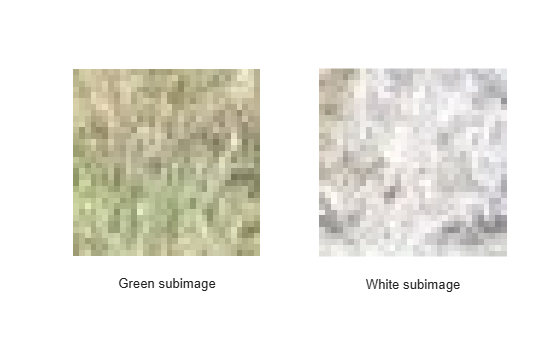Construct FIS

For color segmentation, construct a three-input, one-output Sugeno FIS without rules. For each input and output variable, include two default membership functions (MFs).

colorFISIn = sugfis('NumInputs',3,'NumInputMFs',2, ...

The input variables correspond to the RGB values for each pixel. The output value is high if the pixel color is green; otherwise it is low.

Train FIS

Create training data from the representative color subimages. The getColorInputData helper function, which is shown at the end of the example, creates a three-column array of RGB values for each pixel in a specified image.

[grnSubRow,grnSubCol,grnSubDepth] = size(grnImg);  % Green subimage size
[whtSubRow,whtSubCol,whtSubDepth] = size(whtImg);  % White subimage size
trnX = [...
getColorInputData(grnImg); ...
getColorInputData(whtImg) ...
];
trnY = [...
ones(grnSubRow*grnSubCol,1); ... % Output is high (1) for green pixels
zeros(whtSubRow*whtSubCol,1) ... % Output is low (1) for white pixels
];

Input data trnX has three columns for the RGB pixel values. Output data trnY is a column vector that contains a 1 for each green pixel and a 0 for each white pixel.

Create an option set for learning rules for colorFISIn. To reduce the duration of the optimization process, use the minimum values for cross-validation parameters.

options = tunefisOptions('OptimizationType','learning','KFoldValue',2, ...
'ValidationTolerance',0.0,'ValidationWindowSize',1);

If you have Parallel Computing Toolbox™ software, you can improve the speed of the tuning process by setting options.UseParallel to true. If you do not have Parallel Computing Toolbox software, set options.UseParallel to false.

To learn rules and find FIS parameter values, this example uses genetic algorithm optimization, which is a stochastic process. To obtain reproducible results, initialize the random number generator to its default configuration.

rng('default')

Learn fuzzy rules for colorFISIn using the training data and options. Learning rules using the tunefis function can take several minutes. For this example, you can enable tuning by setting runtunefis to true. To load pretrained results without running tunefis, set runtunefis to false.

runtunefis = false;

To learn new rules without tuning input and output MF parameters, set the parameter settings to []. For more information, see tunefis.

if runtunefis
colorFISOut1 = tunefis(colorFISIn,[],trnX,trnY,options); %#ok<UNRCH>
else
colorFISOut1 = exData.colorFISOut1;
end

Calculate the root mean squared error (RMSE) for the trained FIS. The calculateRMSE helper function, which is shown at the end of the example, classifies the training data pixels using the trained FIS and compares the results to the expected pixel classifications.

fprintf('Training RMSE after learning rules = %.3f MPG\n',...
calculateRMSE(colorFISOut1,trnX,trnY));
Training RMSE after learning rules = 0.283 MPG

After learning the new rules, tune the input and output MF parameters. To obtain the tunable parameter settings of the FIS, use the getTunableSettings function.

[in,out] = getTunableSettings(colorFISOut1);

To tune the existing FIS parameter values without learning new rules, set the OptimizationType to 'tuning'.

options.OptimizationType = 'tuning';

Tune the FIS parameters using the specified tunable settings, training data, and tuning options.

if runtunefis
rng('default')
colorFISOut = tunefis(colorFISOut1,[in;out],trnX,trnY,options);
colorFISOut.Name = "colorFISOut";
else
colorFISOut = exData.colorFISOut;

end

Calculate the RMSE for the tuned FIS.

fprintf('Training RMSE after tuning MF parameters = %.3f MPG\n',...
calculateRMSE(colorFISOut,trnX,trnY));
Training RMSE after tuning MF parameters = 0.228 MPG

Segment Image

Segment the original image using the tuned FIS. To do so, first extract the red, green, and blue pixel values.

[imgRow,imgCol,imgDepth] = size(cImg);
red = cImg(:,:,1);
green = cImg(:,:,2);
blue = cImg(:,:,3);
colorInput = [red(:) green(:) blue(:)];

Classify each pixel using the tuned FIS.

eoptions = evalfisOptions;
eoptions.EmptyOutputFuzzySetMessage = 'none';
eoptions.NoRuleFiredMessage = 'none';
eoptions.OutOfRangeInputValueMessage = 'none';

y = evalfis(colorFISOut,colorInput,eoptions);

Segment the image using the getSegmentedImage helper function, which is shown at the end of the example. This function creates a binary mask from the FIS output values.

greenSegment = getSegmentedImage(reshape(y,[imgRow,imgCol]),cImg);

View the segmented image. Pixels that the FIS classified as white are shown in black. The remaining pixels are classified as green.

figure
imshow(greenSegment)White pixels are partially removed from the border and ball segments. The green segment also incorrectly includes pixels from the ball. Therefore, the classification process requires another pixel attribute that can identify the difference between the grass field and the ball.

Segment Image Using Texture

To distinguish between the field and the ball, use gray image gradient data to identify textures of the field and the ball.

Extract a representative subimage for the ball, and convert the green, white, and ball subimages to grayscale.

ballImg = exData.ballImg;
grayGrnImg = rgb2gray(grnImg);
grayWhtImg = rgb2gray(whtImg);
grayBallImg = rgb2gray(ballImg);

Compute the gradient for each subimage and normalize the gradient magnitude for each pixel using the normMat helper function.

View the gradients for each subimage.

figure,
subplot(2,3,1)
imshow(grnImg)
subplot(2,3,2)
imshow(whtImg)
subplot(2,3,3)
imshow(ballImg)
subplot(2,3,4)
imshow(grnGrsTexture)
subplot(2,3,5)
imshow(whtGrsTexture)
subplot(2,3,6)
imshow(ballTexture)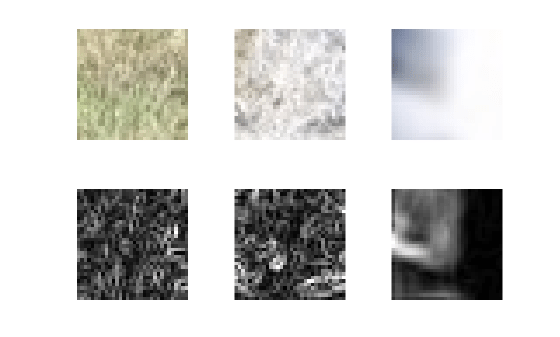Both the green and white grass segments have similar gradient values, which are different than those of the ball segment. Therefore, use only the green and ball segment gradient data to train a fuzzy texture classifier.

Construct FIS

The normalized gradients for the ball and grass field have different patterns. To learn these patterns, create a three-input, one-output Sugeno FIS without rules. For each input and output variable, include two default membership functions (MFs).

textureFISIn = sugfis('NumInputs',3,'NumInputMFs',2, ...

The input variables specify gradient values for three successive pixels. The output value is high if the third pixel belongs to the grass field; otherwise, it is low.

Train FIS

Create training data from the gradients of the green and ball regions. The getGradientInputData helper function, which is shown at the end of the example, creates a three-column array of successive pixel value combinations.

trnX = [...
];
trnY = [...
];

Input data trnX is has three columns for the gradient values of the three successive pixels. Output data trnY is a column vector that contains a 1 if the third pixel belongs to field texture and a 0 otherwise.

To learn fuzzy rules, set the OptimizationType to 'learning'.

options.OptimizationType = 'learning';

Train textureFISIn to learn rules using the training data.

if runtunefis
rng('default')
textureFISOut1 = tunefis(textureFISIn,[],trnX,trnY,options); %#ok<UNRCH>
else
textureFISOut1 = exData.textureFISOut1;
end
fprintf('Training RMSE after learning rules = %.3f MPG\n',...
calculateRMSE(textureFISOut1,trnX,trnY));
Training RMSE after learning rules = 0.477 MPG

After learning the new rules, tune the input and output MF parameters. To obtain the tunable parameters of the FIS, use the getTunableSettings function.

[in,out] = getTunableSettings(textureFISOut1);

To tune the existing FIS parameters without learning new rules, set the OptimizationType to 'tuning'.

options.OptimizationType = 'tuning';

Tune the FIS parameters using the specified tunable settings, training data, and tuning options.

if runtunefis
rng('default')
textureFISOut = tunefis(textureFISOut1,[in;out],trnX,trnY,options);
textureFISOut.Name = "textureFISOut";
else
textureFISOut = exData.textureFISOut;
end
fprintf('Training RMSE after tuning MF parameters = %.3f MPG\n',...
calculateRMSE(textureFISOut,trnX,trnY));
Training RMSE after tuning MF parameters = 0.442 MPG

Segment Image

Segment the original image using the tuned FIS. To do so, first compute the image gradient and extract the successive pixel combinations.

Classify each pixel using the tuned FIS.

Segment the image using the getSegmentedImage helper function.

grassField = getSegmentedImage(reshape(y,[imgRow,imgCol]),cImg);

View the segmented image. Pixels that the FIS classified as belonging to the ball are shown in black. The remaining pixels are classified as field pixels.

figure
imshow(grassField)The trained FIS segments the grass field and the ball with few incorrect pixels in the segments.

Segment Image Using Both Color and Texture

To classify pixels based on both color and texture, you can combine colorFISOut and textureFISOut using a hierarchical fuzzy system, or FIS tree.

To do so, first create a Sugeno FIS with two inputs and three outputs. The first input variable is the output of colorFISOut and the second input variable is the output of textureFISOut. The output variables are the degree to which a pixels belongs to each image segment: green field, white border, and soccer ball.

segFIS = sugfis('Name','segFIS','NumInputs',2,'NumInputMFs',2, ...

Name the input variables, output variable, and MFs.

segFIS.Inputs(1).Name = 'color';
segFIS.Inputs(1).MembershipFunctions(1).Name = 'white';
segFIS.Inputs(1).MembershipFunctions(2).Name = 'green';
segFIS.Inputs(2).Name = 'texture';
segFIS.Inputs(2).MembershipFunctions(1).Name = 'ball';
segFIS.Inputs(2).MembershipFunctions(2).Name = 'grass';
segFIS.Outputs(1).Name = 'greenField';
segFIS.Outputs(1).MembershipFunctions(1).Name = 'low';
segFIS.Outputs(1).MembershipFunctions(2).Name = 'high';
segFIS.Outputs(2).Name = 'whiteBorder';
segFIS.Outputs(2).MembershipFunctions(1).Name = 'low';
segFIS.Outputs(2).MembershipFunctions(2).Name = 'high';
segFIS.Outputs(3).Name = 'soccerBall';
segFIS.Outputs(3).MembershipFunctions(1).Name = 'low';
segFIS.Outputs(3).MembershipFunctions(2).Name = 'high';

Add the following rules to the FIS.

• If the pixel has a smooth ball texture, set the soccer ball output to high.

• If the pixel is white and has a grass texture set the white border output to high.

• If the pixel is green and has a grass texture and is green field output to high.

rules = ["texture==ball => greenField=low, whiteBorder=low, soccerBall=high";
"color==white & texture==grass => greenField=low, whiteBorder=high, soccerBall=low";
"color==green & texture==grass => greenField=high, whiteBorder=low, soccerBall=low"];

Create a FIS tree by connecting the outputs of colorFISOut and textureFISOut to the inputs of segFIS.

fis = [colorFISOut textureFISOut segFIS];
con = [...
"colorFISOut/output1" "segFIS/color"; ...
"textureFISOut/output1" "segFIS/texture" ...
];
fisT = fistree(fis,con);

Classify the image pixels using the FIS tree and segment the image. For each segmented image, the nonblack pixels are classified as part of the segment.

greenField = getSegmentedImage(reshape(y(:,1),[imgRow,imgCol]),cImg);
whiteBorder = getSegmentedImage(reshape(y(:,2),[imgRow,imgCol]),cImg);
soccerBall = getSegmentedImage(reshape(y(:,3),[imgRow,imgCol]),cImg);

View the green field pixels.

figure
imshow(greenField)
xlabel('Green field')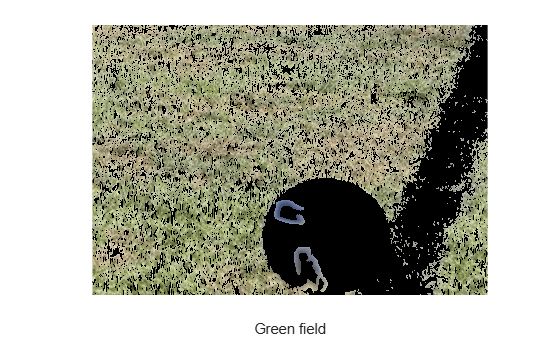View the white border pixels.

figure
imshow(whiteBorder)
xlabel('White border')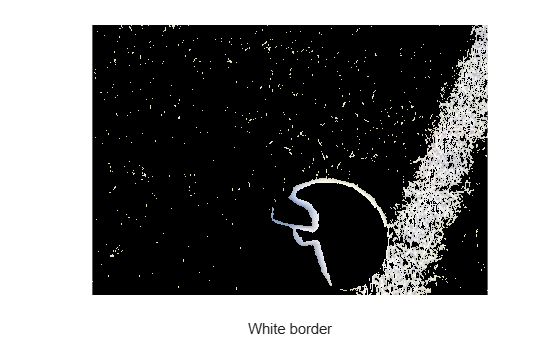View the soccer ball pixels.

figure
imshow(soccerBall)
xlabel('Soccer ball')Conclusion

The image segments contain incorrect classifications. You can remove many of the misclassified pixels by post-processing the results using noise reduction algorithms, such as morphological operations (imdilate, imerode, imopen, imclose). For example, use a morphological close operation to reduce the noise in the green field segmented image.

greenFieldLowNoise = getSegmentedImageClose(reshape(y(:,1),[imgRow,imgCol]),cImg);
figure
imshow(greenFieldLowNoise)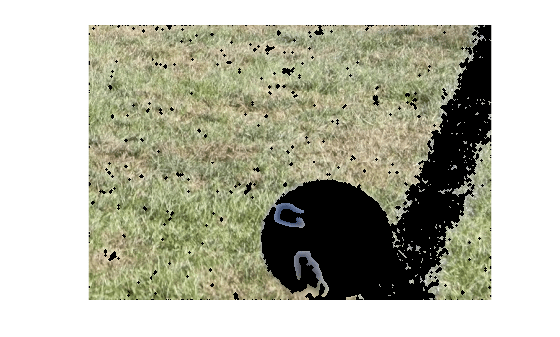To improve fuzzy classifier performance, you can:

• Use more training data.

• Learn color patterns of multiple pixels instead of learning individual pixel color.

• Increase the length of the gradient feature vector, in other words, use gradient values of more than three successive pixels.

• Add more MFs to the FIS for pixel classification.

• Use type-2 FIS.

• Use a validation tolerance, a larger window size, and higher k-fold values for cross validation.

• Tune the parameters of the constructed FIS tree segFIS.

Local Functions

function data = getColorInputData(img)
% Create RGB input data from an image for training.

[row,col,depth] = size(img);
data = zeros(row*col,depth);
id = 0;
for i = 1:row
for j = 1:col
id = id + 1;
for k = 1:depth
data(id,k) = img(i,j,k);
end
end
end

end

function [rmse,actY] = calculateRMSE(fis,x,y)
% Calculate root mean squared error for FIS output.

% Specify options for FIS evaluation
persistent evalOptions
if isempty(evalOptions)
evalOptions = evalfisOptions("EmptyOutputFuzzySetMessage","none", ...
"NoRuleFiredMessage","none","OutOfRangeInputValueMessage","none");
end

% Evaluate FIS
actY = evalfis(fis,x,evalOptions);

% Calculate RMSE
del = actY - y;
rmse = sqrt(mean(del.^2));

end

function cImg = getSegmentedImage(y,cImg)
% Segment an image using classifier output by creating a binary image
% using a 0.5 threshold.

id = y >= 0.5;
y(id) = 1;
y(~id) = 0;

cImg(:,:,1) = cImg(:,:,1).*y;
cImg(:,:,2) = cImg(:,:,2).*y;
cImg(:,:,3) = cImg(:,:,3).*y;

end

function y = normMat(x)
% Normalize array elements to the range [0 1].

tmp = x(:);
mn = min(tmp);
mx = max(tmp);
d = (mx-mn);
y = (x-mn);
if d>0
y = y/d;
end

end

% Create gradient input data for training.

x = x(:);
n = 3; % Three successive gradient values.
data = zeros(length(x),n);

% Specify complete input vectors.
for i = n:length(x)
data(i,:) = x(i-n+1:i)';
end

% Approximate missing elements in the incomplete input vector.
for i = n-1:-1:1
right = x(1:i)';
m = n - i;
left = repmat(right(1),[1 m]);
data(i,:) = [left right];
end

end

function cImg = getSegmentedImageClose(y,cImg)
% Segment an image using classifier output by creating a binary image
% using a 0.5 threshold.

id = y >= 0.5;
y(id) = 1;
y(~id) = 0;

se = strel('disk',1);
y = imclose(y,se);

cImg(:,:,1) = cImg(:,:,1).*y;
cImg(:,:,2) = cImg(:,:,2).*y;
cImg(:,:,3) = cImg(:,:,3).*y;

end